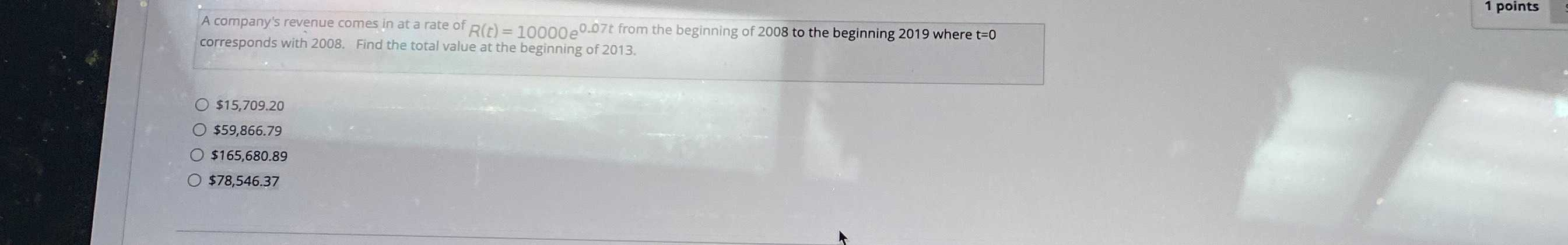### ¿Todavía tienes preguntas de matemáticas?

Pregunte a nuestros tutores expertos
Algebra
PreguntaA company's revenue comes in at a rate of $$R ( t ) = 10000 e ^ { 0.07 t }$$ from the beginning of $$2008$$ to the beginning $$2019$$ where $$t = 0$$ corresponds with $$2008$$ . Find the total value at the beginning of $$2013$$ .

$$\ 15,709.20$$

$$\ 59,866.79$$

$$\ 165,680.89$$

$$\ 78,546.37$$